## 4.3 Base R graphics

This chapter covers base R graphics, rather than ggplot2, which is covered in Chapter 2: Visualizing data of the ds4psy textbook.

Our main goals are:

• Hands-on instructions on visualizing data in base R.

• Distinguishing between different types of visualizations.

• Adding aesthetics: Color, shape, size, etc.

Two important caveats:

1. Graphs often requires transforming data into a specific format or shape. We ignore this here, but will return to this topic in our part on wrangling data.

2. We occasionally use colors, but do not cover how to specify and select colors in R. In the following, we occasionally select colors and color functions of the unikn package (see Appendix D: Using colors for details).

### 4.3.1 Basic plots

Due to the inclusion of the core packages graphics and grDevices, a standard installation of R comes with pretty powerful tools for creating a variety of visualizations.

In this chapter, we can only introduce some very basic commands for creating typical (named) graphs. We can distinguish between basic and complex plots:

• Basic plots create an entire plot in one function call:
• hist() creates histograms;
• plot() creates point and line plots (as well as more complex ones);
• barplot() creates bar charts;
• boxplot() creates box plots; and
• curve() allows drawing arbitrary lines and curves.

Complex plots (discussed below) are created by multiple function calls. They are typically started with a generic call to:

• plot(), and then followed by more specific plot functions, like
• grid(), abline(), points(), text(), title(), etc.

#### Histograms

Histograms are one of the simplest types of plots: They show the distribution of values of a single variable.

For demonstration purposes, we create a vector x that randomly draws 500 values of a normal distribution:

v <- rnorm(n = 500, mean = 100, sd = 10)

In R, the hist() function allows specifying the data to plot as an argument x. Providing our vector v to the function yields the following:

hist(x = v)This looks fairly straightforward, but due to the random nature of x the distribution of its values will vary every time we re-create the vector x.

Note that the hist() command added some automatic elements to our plot:

• a descriptive title (above the plot);
• an x- and a y-axes, with appropriate value ranges, axis ticks and labels;
• some geometric objects (here: vertical bars or rectangles) that represent the values of data.

Under the hood, the function even re-arranged the input vector and computed something: It categorized the values of x into bins of a specific width and counted the number of values falling into each bin (to show their frequency on the y-axis).

Whenever we are unhappy with the automatic defaults, we can adjust some parameters. In the case of histograms, an important parameter is the number of separate bins into which the data values are being categorized. This can be adjusted using the breaks argument:

# specifying breaks:
hist(v, breaks =  5)hist(v, breaks = 25)Once we have settled on the basic parameters, we can adjust the labels and aesthetic aspects of a plot. A good plot should always contain informative titles, value ranges, and labels. In the following expression, we adjust the main title (using the main argument), the label of the x-Axis (using xlab argument), the main color of the bars and their border (using the col and border arguments):

# with aesthetics:
hist(v, breaks = 20,
main = "A basic histogram (showing the distribution of x)",
xlab = "Values of x",
col = "gold", border = "blue")Note that we did not adjust the range of values on the x- and y-axes. If we wanted to do this, we could have done so by providing the desired ranges (each as a vector with two numeric values) to the xlim and ylim arguments:

# with aesthetics:
hist(v, breaks = 20,
main = "A basic histogram (showing the distribution of x)",
xlab = "Values of x",
col = "gold", border = "maroon",
xlim = c(50, 150), ylim = c(0, 120))#### Scatterplots

The plot() function shows relationships between two variables x and y. Actually, plot() is a flexible plotting function in R: On the one hand, it allows defining new plots (e.g., create a new plotting canvas). On the other hand, calling the function with some data arguments aims to directly create a plot of them.

In this section, we will call it with two vectors x and y to create a scatterplot (i.e., a plot of points). However, we will also see that plot() allows creating different plots of the same data, specifically:

• a line plot;
• a step function.

We first create some data to plot. Here are two simple numeric vectors x and y (where y is defined as a function of x):

# Data to plot:
x <- -10:10
y <- x^2  

When providing these vectors x and y to the x and y arguments of the plot() function, we obtain:

# Minimal scatterplot:
plot(x = x, y = y)Thus, the default plot chosen by plot() was a scatterplot (i.e., a plot of points). We can change the plot type by providing different values to the type argument:

# Distinguish types:
plot(x, y, type = "p")  # points (default)plot(x, y, type = "l")  # linesplot(x, y, type = "b")  # both points and linesplot(x, y, type = "o")  # both overplottedplot(x, y, type = "h")  # histogram or height densityplot(x, y, type = "s")  # stepsSee the documentation ?plot() for details on these types and additional arguments. For most datasets, only some of these types make sense. Actually, one of the most common uses of plot() uses the type n (for “no plotting”):

plot(x, y, type = "n")  # no plotting / nothingAs we see, this does not plot any data, but created an empty plot (with appropriate axis ranges). When creating more complex plots (below), we start like this and then add various custom objects to our plot.

Once we have selected our plot, we can fiddle with its aesthetic properties and labels to make it both prettier and more informative:

# Set aesthetic parameters and other options:
plot(x, y, type = "b",
lty = 2,  pch = 16, lwd = 2, col = "red", cex = 1.5,
main = "A basic plot (showing the relation between x and y)",
xlab = "X label", ylab = "Y label",
asp = 1/10  # aspect ratio (x/y)
)#### Overplotting

A common problem with scatterplots is overplotting (i.e., too many points at the same locations). For instance, suppose we wanted to plot the following data points:

# Data to plot:
x <- runif(250, min = 0, max = 10)
y <- runif(250, min = 0, max = 10)

Here is how basic scatterplot (with filled and fairly large points) would look like:

# Basic scatterplot:
plot(x, y, type = "p",
pch = 20, cex = 4, # filled circles with increased size
main = "An example of overplotting")Note: In case you’re wondering what pch and cex mean:

• Calling ?par() provides detailed information on a large variety of graphical parameters.
• Calling par() shows your current system settings.

One of several solutions to the overplotting problem lies in using transparent colors, that allow viewing overlaps of graphical objects. There are several ways of obtaining transparent colors. For instance, the following solution uses the unikn package to select some dark color (called Petrol) and then use the usecol() function to set it to 1/3 of its original opacity:

library(unikn)
my_col <- usecol(Petrol, alpha = 1/3)

Providing my_col to the col argument of plot() yields:

# Set aesthetic parameters and other options:
plot(x, y, type = "p",
pch = 20, cex = 4,
col = my_col,
main = "Addressing overplotting (by color transparency)")Note that the following type variants of plot() may look pretty, but unless we are trying to make an artistic point they make very limited sense given this data:

# Select colors:
my_cols <- usecol(c(Karpfenblau, Pinky, Petrol, Bordeaux), alpha = 2/3)

# Plot 4 types of same data:
plot(x, y, type = "l", col = my_cols, main = "A: Line plot")
plot(x, y, type = "b", col = my_cols, main = "B: Both points and lines")
plot(x, y, type = "h", col = my_cols, main = "C: Height density plot")
plot(x, y, type = "s", col = my_cols, main = "D: Step plot")Figure 4.2: Plot types not suited for the current data.

Thus, which type of plot() makes sense is primarily a function of the data that is to be shown.1

#### Bar plots

One of the most common, but also quite complicated types of plots are bar plots (aka. bar charts). The two main reasons why bar plots are complicated are:

1. the bars often represent processed data (e.g., counts, or the means, sums, or proportions of values).

2. the bars can be arranged in multiple ways (e.g., stacked vs. beside each other, grouped, etc.)

When we have a named vector of data values that we want to plot, the barplot() command is pretty straightforward:

# A vector as data:
v <- c(1, 3, 2, 4, 2)        # some values
names(v) <- c(LETTERS[1:5])  # add names

barplot(height = v, col = Seeblau)In most cases, we have some more complicated data (e.g., a data frame or multiple vectors). To create a bar graph from data, we first create a table that contains the values we want to show.

A simple example could use the mpg data from ggplot2:

# From data:
mpg <- ggplot2::mpg

# Turn class into a factor variable:
mpg$class <- factor(mpg$class)

The following table() function creates a simple table of data by counting the number of observations (here: cars) for each level of the class variable:

# Create a table:
tb <- table(mpg$class) # names(tb) tb ## ## 2seater compact midsize minivan pickup subcompact suv ## 5 47 41 11 33 35 62 Providing this table as the height argument of barplot() creates a basic bar plot: barplot(height = tb) # basic versionAdding aesthetics and labels renders the plot more colorful and informative: barplot(height = tb, main = "Counts of cars by class", xlab = "Class of car", las = 2, # vertical labels col = usecol(pal_unikn_light))An alternative way of creating a barplot() would use the data and formula arguments: Using the UCBAdmissions data: df <- as.data.frame(UCBAdmissions) df_A <- df[df$Dept == "A", ]
df_E <- df[df$Dept == "E", ] # Select 2 colors: my_cols <- c(Seeblau, Bordeaux) # two colors Create two bar plots: # A: barplot(data = df_A, Freq ~ Gender + Admit, beside = TRUE, main = "Department A", col = my_cols, legend = TRUE)# E: barplot(data = df_E, Freq ~ Gender + Admit, beside = TRUE, main = "Department E", col = my_cols, legend = TRUE)Problem: Legend position overlaps with bars. Two possible solutions: # Moving legend position: # Solution 1: specify args.legend (as a list) barplot(data = df_E, Freq ~ Gender + Admit, beside = TRUE, main = "Department E", col = my_cols, legend = TRUE, args.legend = list(bty = "n", x = "topleft"))# Solution 2: Adjust the size of the x-axis: barplot(data = df_E, Freq ~ Gender + Admit, beside = TRUE, main = "Department E", col = my_cols, legend = TRUE, xlim = c(1, 8))### 4.3.2 Complex plots The commands covered so far provide shortcuts and all do multiple things (e.g., select default layouts, range and labels on axes, as well as drawing particular objects). If we want more control about our plots or modify some of the automatic choices, we can specify all parts of a plot in detail. Complex plots are created by multiple function calls and typically start by calling plot() with type = "n" to create a basic plot or canvass, and then adding additional objects to it. More specific plot functions include grid(), abline(), points(), text(), title(), as well as setting graphical parameters (with par()). In the following, we will illustrate the most important functions in the context of some visualizations. #### Composing plots as programs More advanced plots in the base R plotting system are created by calling successive R functions to incrementally build a visualization. When constructing more advanced visualizations, plotting occurs in two main stages: 1. Calling plot() for creating and defining the dimensions of a new plot; 2. Using annotation functions for adding elements to the plot (e.g., points, lines, or text elements, as well as grids, titles, labels, and legends). This two-stage process essentially turns the holistic task of “making a plot” into one of step-wise planning and composition. Rather than just calling one command that creates the entire plot, we start with a simple and reduced version (think: a blank canvas) and then use the tools provided by R (think: brushes, color, shapes, etc.) to improve our visualization. If we include the preparatory steps that precede plotting, the process of creating a visualization is structured like any other computer program: 1. Define data objects and other elements to be used (e.g., the values of parameters, colors), 2. Prepare the plotting canvas (dimensions, axes), 3. Add objects by calling functions that create new objects. Note that — just as in other programs — the order of operations matters. When creating complex graphs, this is true in both a logical and a visual sense: We generally need to define an object (e.g., a value or color) prior to using it in an evaluated expression or function. But when creating a visualization and wanting to show some object A in front of another object B, we need to draw B before drawing A (so that A is plotted on top of B). #### Starting a new plot We have seen above that the plot() function can create a scatterplot, or other types of plot depending on the type of object being plotted. However, plot() can also be used to merely create a blank plot (i.e., start a new screen device, e.g., in the Plots window of RStudio) to which we then add the elements of our graph. To understand this process, evaluate and compare the result of the following commands: plot(x = 0) plot(x = 0, type = "n")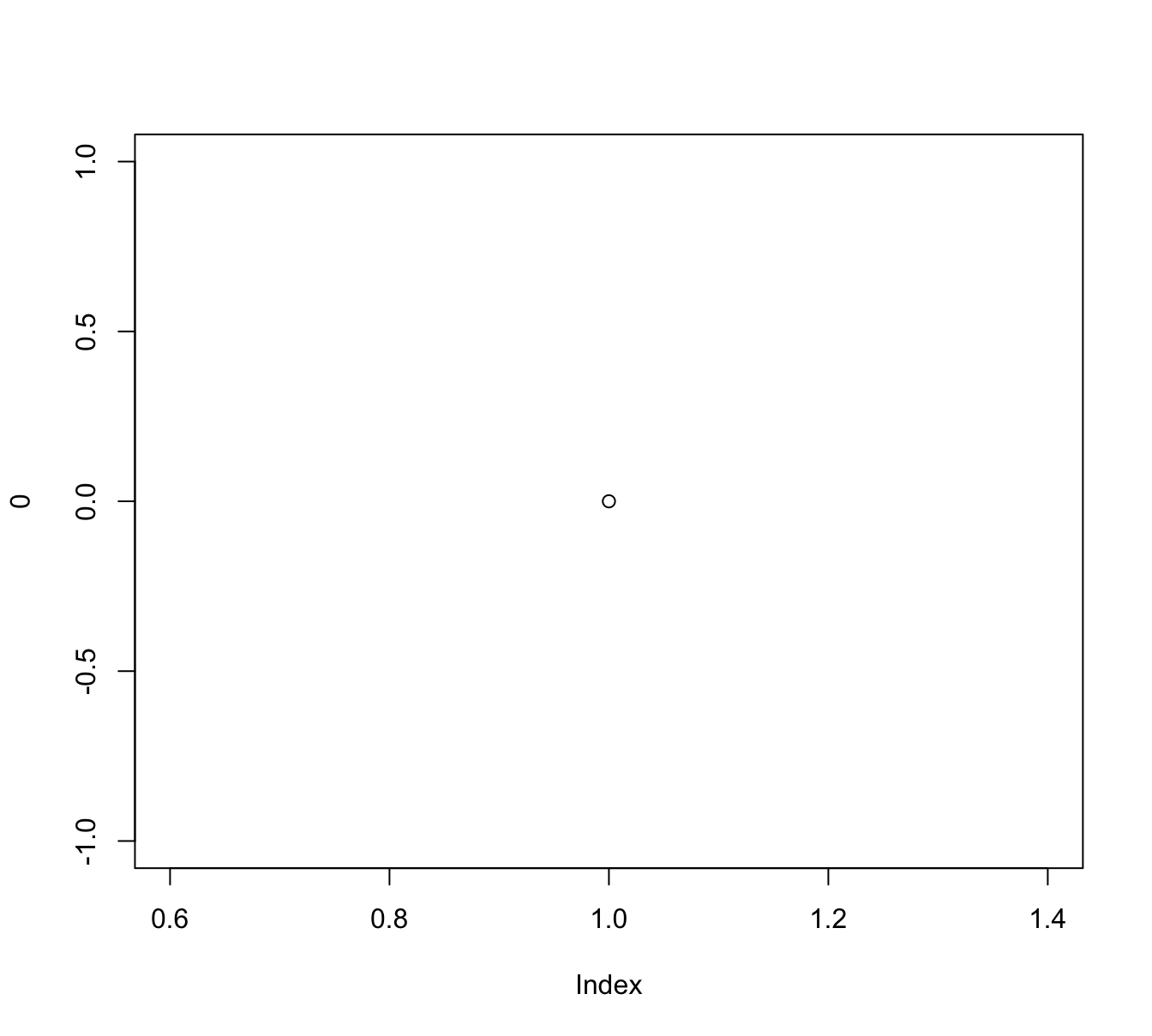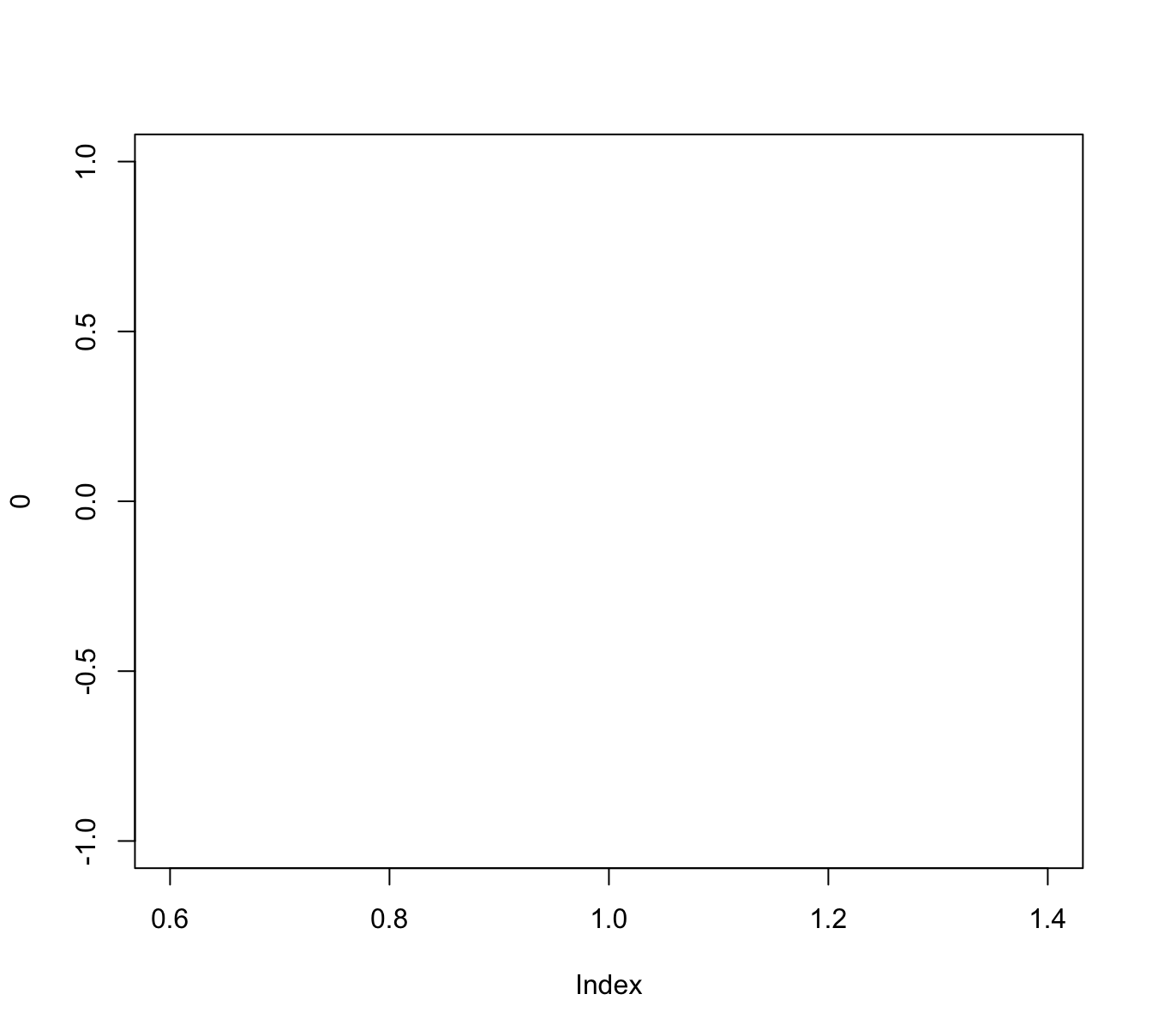We see that both commands create a new plot, but plot(x = 0) creates a scatterplot of a single point (with coordinates of $$(0, 0)$$) whereas adding the argument type = "n" instructed R to merely define a new plotting canvas. However, the new plot still used x to choose ranges for both axes. If we want different ranges, we either can change our data inputs (to x and/or y) or explicitly control the ranges of axes: # (a) use data to define ranges: xs <- -10:10 ys <- xs^2 plot(x = xs, y = ys, type = "n") # (b) define ranges without data: plot(x = 0, xlim = c(-10, 10), ylim = c(0, 100), type = "n")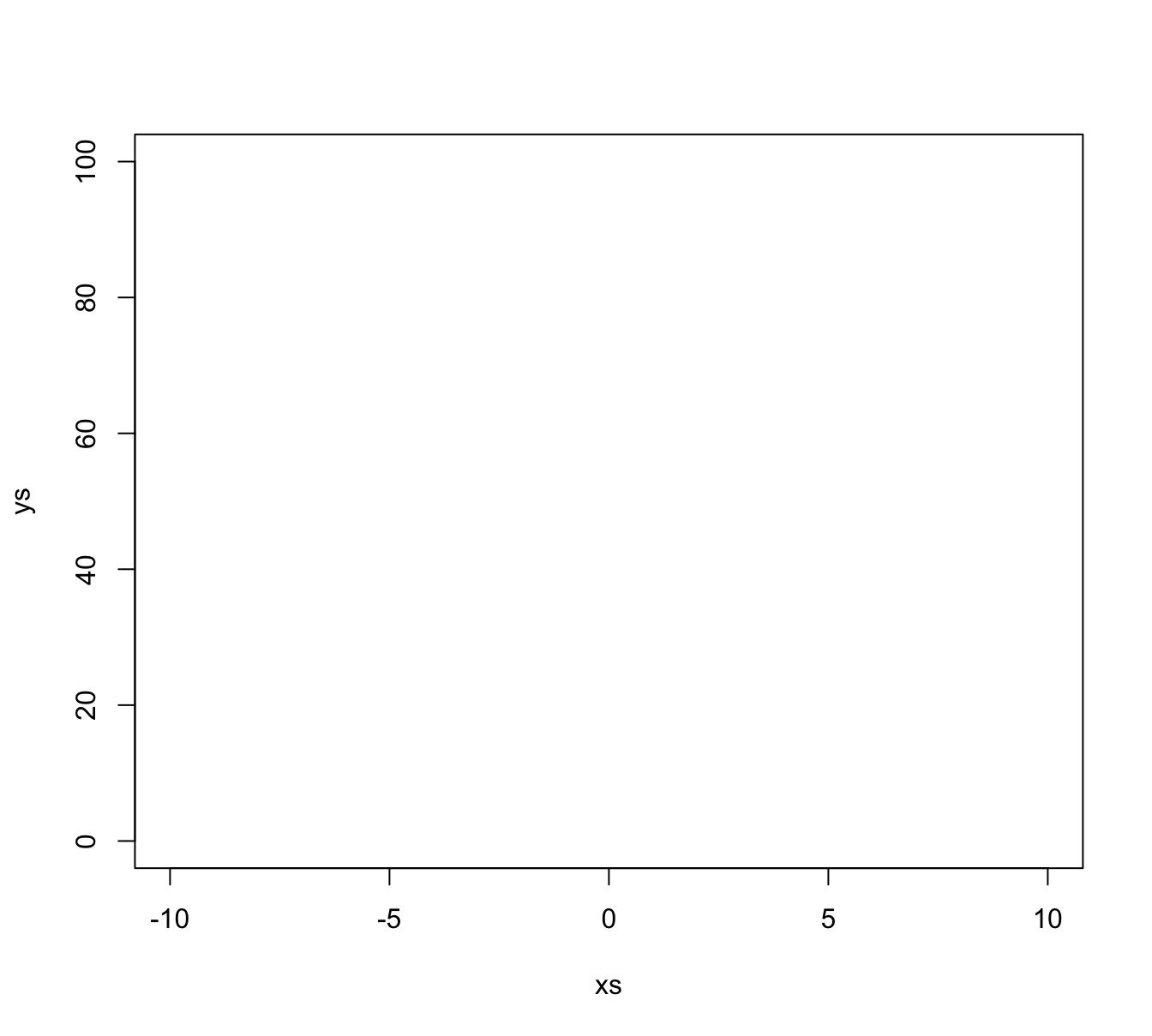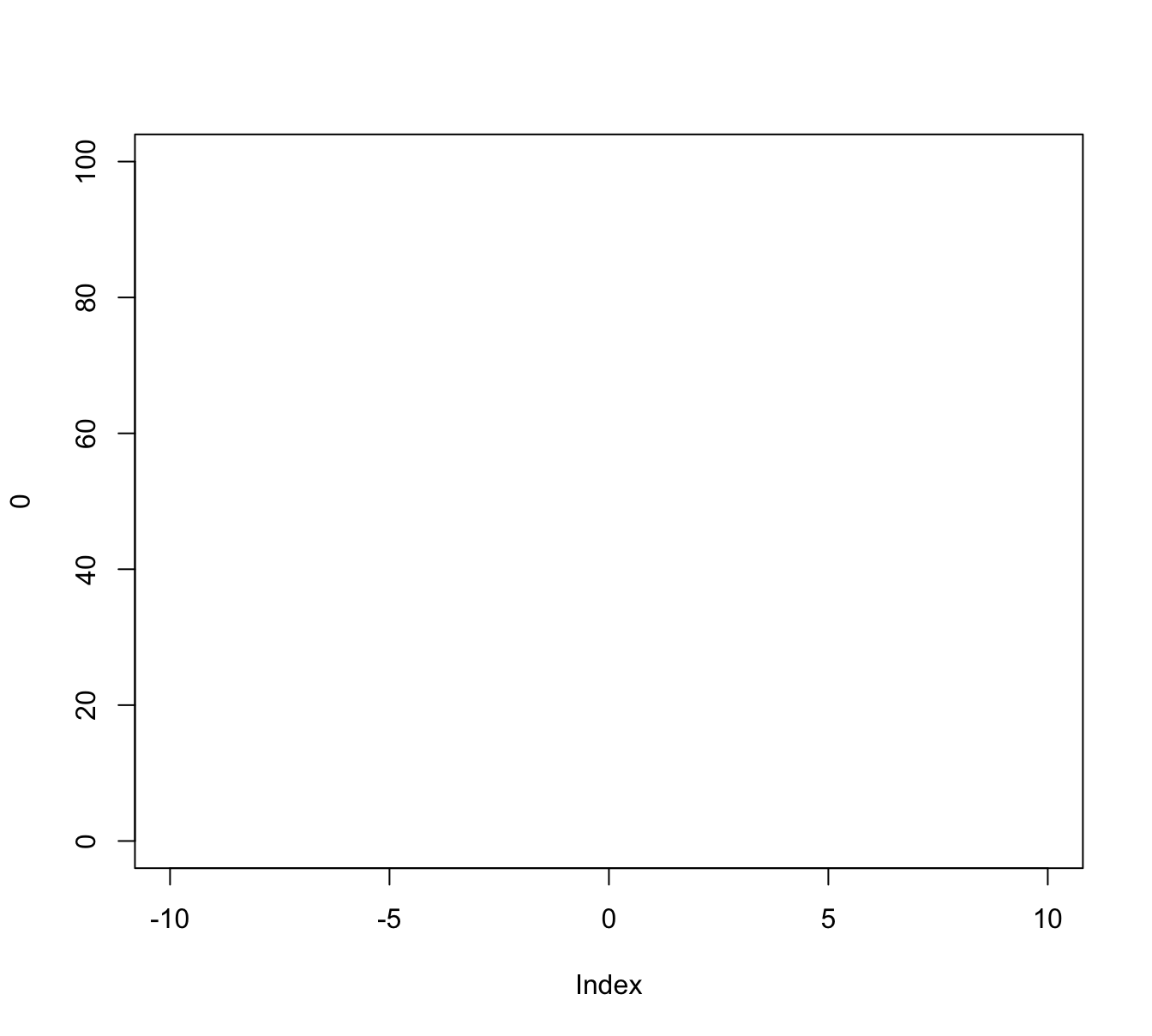Note that both plots just created use the same ranges, but differ in the labels used on the x- and y-axes. If we wanted to control these manually, we could have used the xlab and ylab arguments of plot(). #### Annotating a plot Once we have created a new screen device and defined the dimensions of our plotting region, we can use so-called annotation functions to add to an existing plot. Some key annotation functions include: • points() adds points (or circles) to a plot • abline(), lines(), and segments() add various types of lines to a plot (given their x and y coordinates, or defining other properties, like a linear function’s intercept and slope) • rect() adds rectangles or boxes (given the coordinates of their corners) • text() adds text labels to a plot (to specific x and y coordinates) Other functions allow adding or editing elements on the plot background, axes, and margins: • axis() adds axis labels and ticks • grid() adds orientation lines • title() adds labels to the axes, title, and subtitle, and outer margin • mtext(): add arbitrary text labels to the plot’s (inner or outer) margins Overall, these and other functions allow the composition of arbitrarily complex visualizations. As an example, the following code illustrates the process of first defining a plotting region and then using functions for adding various points, lines, shapes, and text elements. To really understand this process, it makes sense to evaluate the chunk in a step-by-step fashion (i.e., one command at a time): # (0) Define some colors: ----- library(unikn) # to get color palettes my_colors <- usecol(pal_unikn_light) # seecol(my_colors) # view color palette # (1) Create canvas (but specify dimensions and some labels): ----- plot(x = 0, xlim = c(-10, 10), ylim = c(0, 100), type = "n", main = "Plotting various shapes and text labels", xlab = "X value", ylab = "Y value") # (2) Add lines: ----- abline(h = 50, lty = 2) abline(v = 0, lty = 2) abline(a = 10, b = 10) # (3) Add points (as circles): ----- points(x = c(-5, 0, 5), y = c(40, 50, 60), pch = 21, cex = 20, lwd = 4, col = my_colors[c(1, 3, 5)], bg = usecol("grey", alpha = 1/2)) # (4) Add text labels: ----- text(x = 0, y = 50, labels = "R", cex = 4) text(x = -5, y = 40, labels = "me", cex = 2) text(x = 5, y = 60, labels = "stuff", cex = 2) # (5) Add other shapes: ----- rect(xleft = c(-1, -9), xright = c(7, 1), ybottom = c(0, 80), ytop = c(20, 95), col = usecol(my_colors, alpha = 1), border = my_colors[c(7, 5)], lwd = 4) # (6) More text labels: ----- text(x = -4, y = 87, labels = "R pour l'art", col = Bordeaux, cex = 1.5) text(x = 3, y = 10, labels = "WOW!", col = Karpfenblau, cex = 3) # (7) Add line segments: ----- segments(x0 = c(-4, -4, 5, 9), y0 = c(20, 40, 25, 30), x1 = c(9, 3, -8, -4), y1 = c(35, 85, 60, 80), lwd = 5, col = my_colors)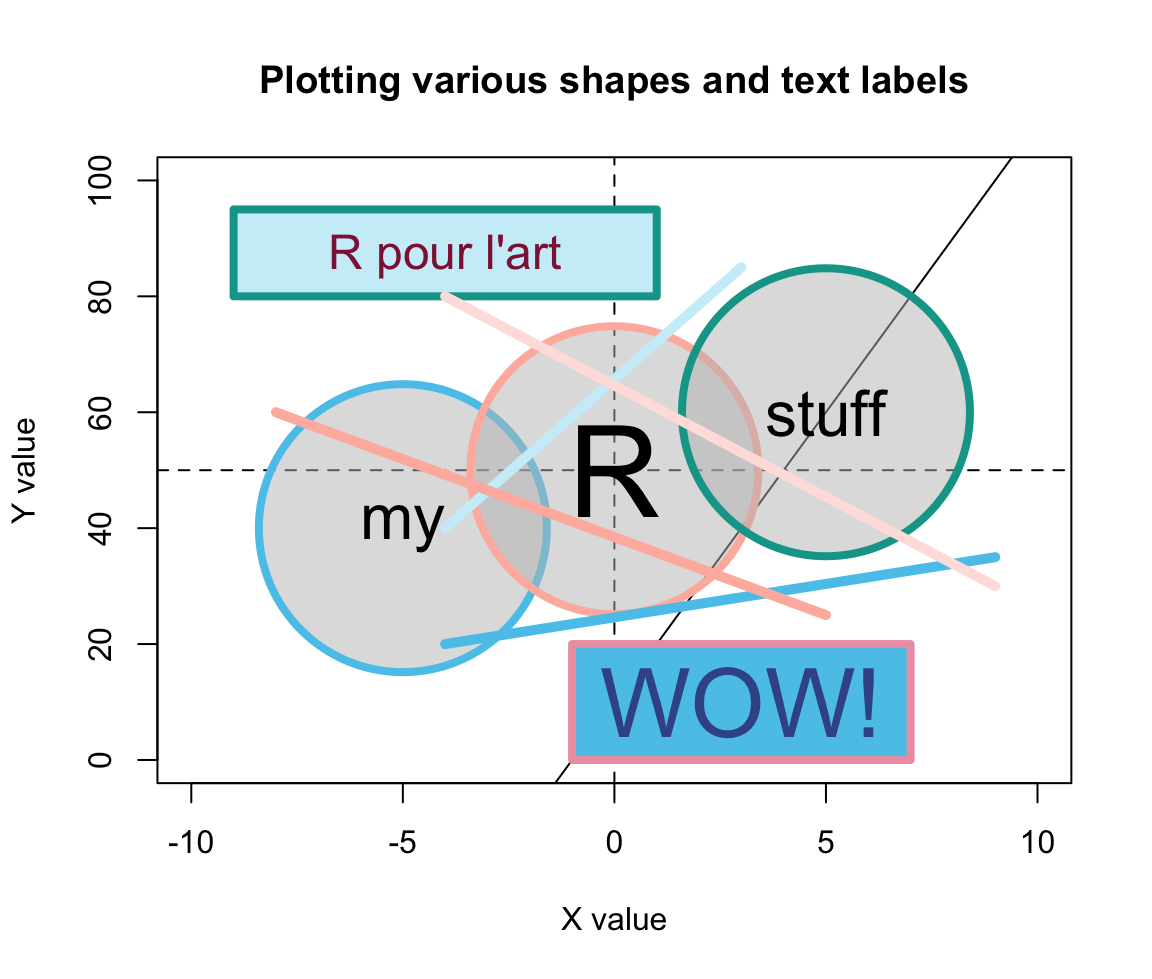Figure 4.3: An example of a complex plot, in which various elements are added to a prepared canvas. Figure 4.3 shows that using transparent colors somewhat tempers the constraints imposed by sequentially plotting objects. For instance, the horizontal, vertical, and diagonal lines created by abline() (in Step 2) are not completely covered by the round shapes (due to setting alpha = 1/2 in their background color bg). By contrast, the two rectangles created by rect() (in Step 5) used a setting of alpha = 1 in their col definition. As a color’s alpha level ranges from 0 to 1 (with 0 indicating complete transprency and 1 indicating no transparency), using alpha = 1 is equivalent to “no transparency” — which is why the rectangles cover the lines created by abline(). Whereas Figure 4.3 arranges graphical elements without a particular purpose, the same methods can be used to produce highly versatile and useful visualizations. For instance, the R package riskyr package provides a range of visualizations that depict the effects of probabilistic binary distinctions based on a population of elements (aka. Bayesian reasoning or diagnostic testing). Here is an example that illustrates the type of problem addressed by such diagrams: • Suppose there is a pandemic (e.g., called COVID-19) that infects 5% of some population of people. • There is a diagnostic screening test with the following properties: • If someone is infected with COVID-19, the tests detects this accurately with a probability of 99%. • If someone is not infected with COVID-19, the tests detects this accurately with a probability of 95%. • Someone receives a positive test result. How likely is it that this individual is infected? In diagnostic terms, the problem specifies the prevalence of some condition (here: 5% of some population are infected with COVID-19) and the sensitivity (99%) and specificity (95%) of some diagnostic test. The problem then asks for the conditional probability of being infected, given a positive test result (which is known as the test’s positive predictive value, PPV). Research has shown that this problem is notoriously difficult, even for medical experts (see REFs for an analysis and meta-review). As the correct answer is the inverse conditional probability of the sensitivity provided (and we are also provided with a base rate/prevalence and the specificity), the answer could be computed using Bayes’ theorem. ToDo: Bayes theorem: Show formula and result: PPV = 51% Note: Given these values, the predictive value of a positive test result (PPV) is about 51%. Thus, throwing a coin is about as accurate as a single result of this test. (Importantly, this does not mean that the test is bad or useless. Things change dramatically if the prevalence of the condition in your environment (which is typically only a sub-part of the population) increased. For instance, if the prevalence of Covid in your environment was 20%, the same test would have a PPV of 83.2%. Alternatively, performing multiple tests with positive results would quickly raise the value of the test results.) However, there are many other ways of computing the correct answer — and most of them involve a representational change in perspective (see Neth et al., 2021, for an analysis). Our goal here is not to solve the problem, but merely to illustrate that the problem could be solved by drawing frequency diagrams — and that we could use base R functions for creating relatively complex diagrams out of lines, shapes, and text labels. For many problems, we do not even need to do this ourselves, as the chances are quite high that someone else has already written an R package that does the job for us. In the current case, the riskyr package illustrates the relationship between a diagnostic test’s accuracy and predictive values in a variety of ways. (Note that you do not need to install the package for yourself, unless you wanted to re-create the plots.)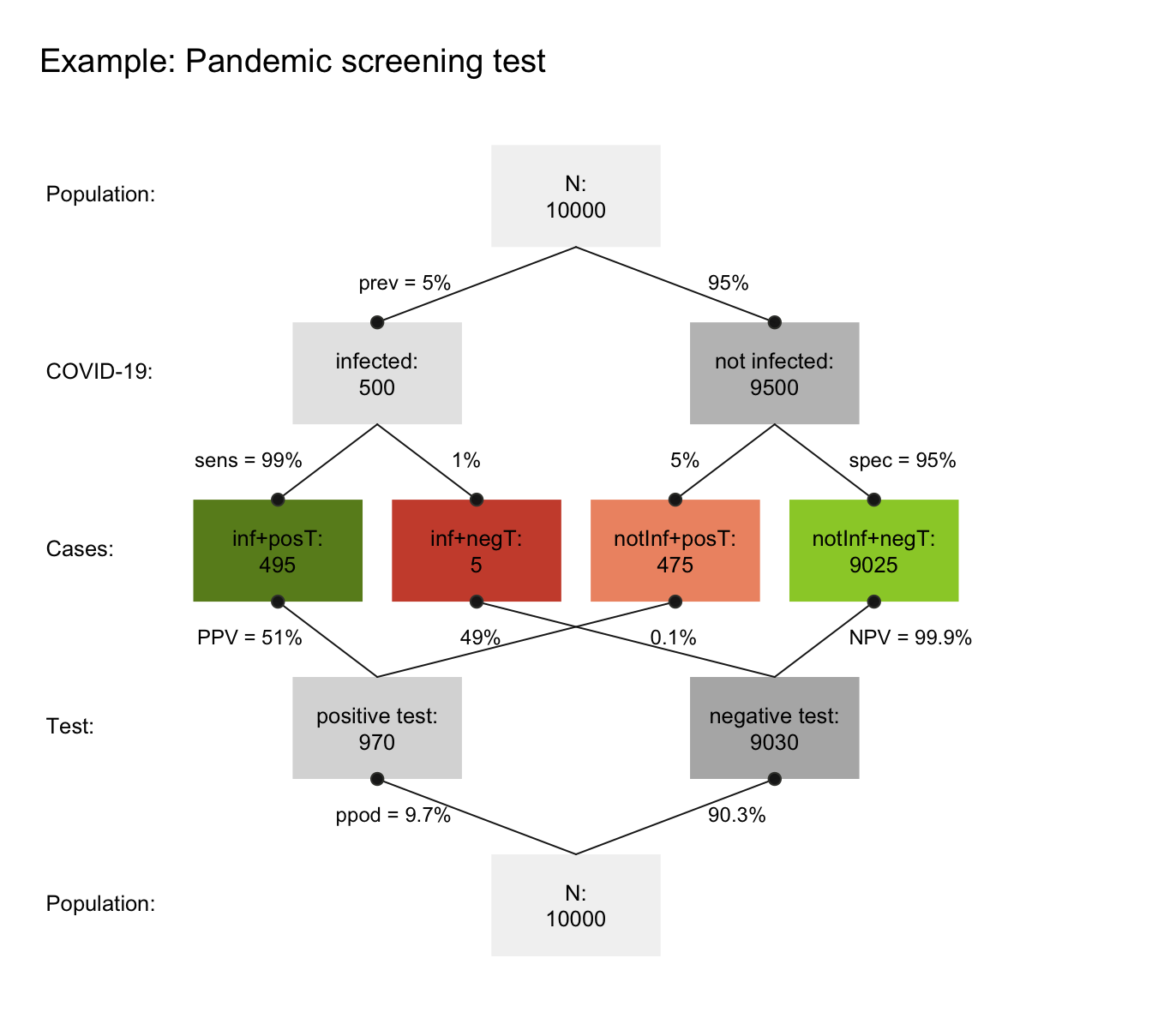Figure 4.4: A prism diagram illustrating the relation between diagnostic accuracy and predictive power of a test (using the riskyr package). Figure 4.4 illustrates the relation between the test’s ability for detecting infected vs. healthy people and its predictive values in a diagram that combines two trees (one top-down, and an inverted one bottom-up). To provide concrete frequencies, both trees assume a fixed population of 10,000 individuals. The top one first dissects the population by condition (cd, i.e., 5% of the people are infected vs. 95% are not infected), then by the specificity and sensitivity of the test. The lower and inverted tree re-combines the four middle cells (in which green cells indicate correct classifications, whereas red ones indicate erroneous classifications) by test result or decision (dc, i.e., positive vs. negative tests). This reveals that the desired probability of being infected, given a positive test result is only $$PPV=51$$% — much lower than most people would expect. Importantly, we could create visualizations like Figure 4.4 from scratch by plotting boxes, lines, and labels. As this would be a lengthy and laborious process, R packages like riskyr facilitate creating various types of plots (see riskyr.org for interactive versions). But it is good to know that we can always create things ourselves, if we had to or wanted to do so. ### 4.3.3 Useful combinations In practice, most R plots require not just one command, but multiple functions. We typically start out with a simple plot and then add or change elements to improve it. Here are some combinations that are quite common and worth knowing: Example 1: A scatterplot with a grouping variable (and legend): The iris data contained in datasets of R contains four types of measurements of three Species of flowers. Suppose we wanted to plot the relationship between Sepal.Length and Petal.Length as a scatterplot. The following plot would reveal a positive correlation between both measurements: plot(x = iris$Sepal.Length, y = iris$Petal.Length, # x and y variables pch = 16, cex = 2, # aesthetic parameters xlab = "Sepal Length", ylab = "Petal Length", # axis labels main = "Flower characteristics in Iris") # title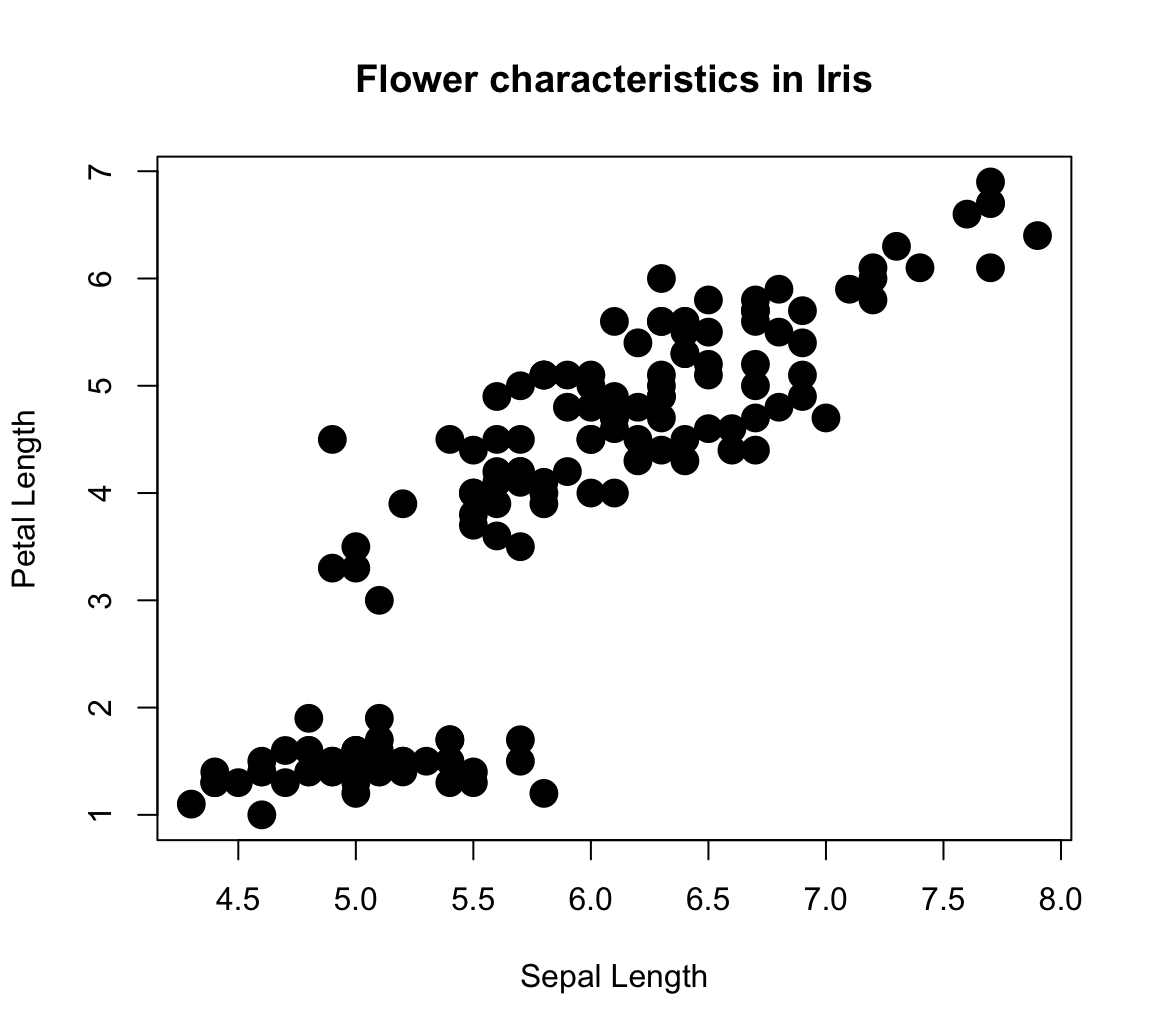However, the plot is deficient in an important respect: We lack all information regarding the Species of each point (which happens to be a factor variable of the iris data). An interesting feature of scatterplots is that we can assign its col aesthetic to a factor variable (i.e., internally encoded by a different integer value for each level of a categorical variable). The R plotting system uses a color vector palette() as its default colors. Setting col = iris$Species essentially instructs R to use the 1st color of palette() for the 1st species, the 2nd color of palette() for the 2nd species, etc.

plot(x = iris$Sepal.Length, y = iris$Petal.Length,  # x and y variables
col = iris$Species, # color by species! pch = 16, cex = 2, # aesthetic parameters xlab = "Sepal Length", ylab = "Petal Length", # axis labels main = "Flower characteristics in Iris") # title # Adding grid: grid() # Adding a legend: legend (x = 4.5, y = 7, legend = levels(iris$Species),
pch = 16, col = c(1:3))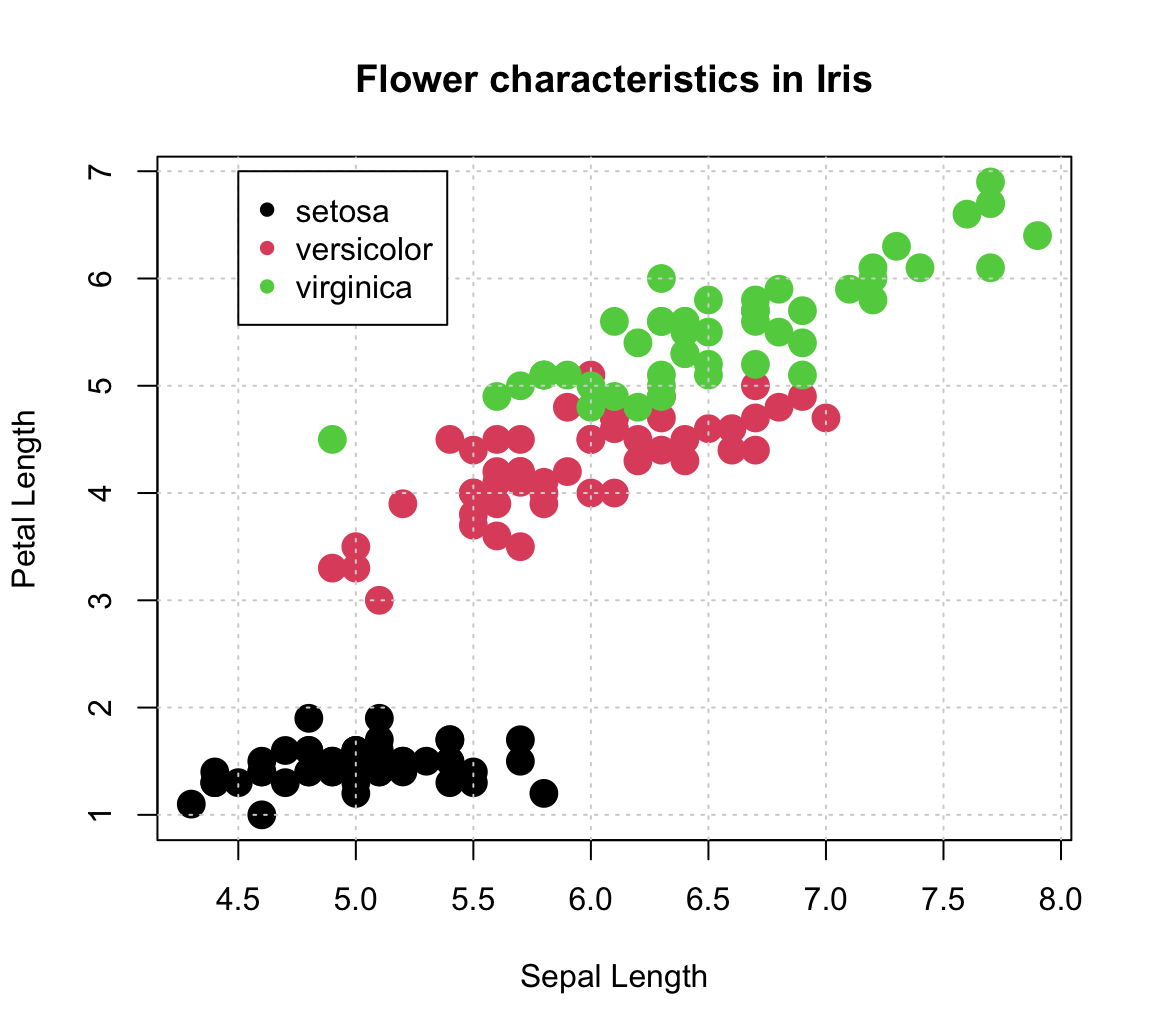Note that we used the legend() function to explicate the mapping of colors to species. The function’s x- and y-coordinates placed its top-left corner inside the plot, but its two key arguments were:

• legend to print the levels of iris$Species (as a character vector); • col explicitly set to the first three colors of the default palette(). Defining another color palette: # (a) Default (base R): palette() # show current default colors ##  "black" "#DF536B" "#61D04F" "#2297E6" "#28E2E5" "#CD0BBC" "#F5C710" ##  "gray62" # Set to different values: palette(rainbow(4)) # use color function palette(c("steelblue", "gold", "firebrick", "forestgreen")) # use color() names # (b) Use hcl color palettes: # hcl.pals() palette(hcl.colors(3, "Red-Blue")) palette(hcl.colors(3, "Viridis")) # (c) color packages: library(unikn) palette(usecol(pal_unikn_pref)) # use color function with palette palette(c(Seeblau, Pinky, Seegruen)) # use color names palette(usecol(c(Seeblau, Pinky, Seegruen), alpha = 2/3)) # by names, plus transparency # palette("default") # reset to default color palette Adding a linear regression line: plot(x = iris$Sepal.Length, y = iris$Petal.Length, # x and y variables col = iris$Species,                            # color by species!
pch = 16, cex = 2,                             # aesthetic parameters
xlab = "Sepal Length", ylab = "Petal Length",  # axis labels
main = "Flower characteristics in Iris")       # title

grid()

legend (x = 4.5, y = 7, legend = levels(iris$Species), pch = 16, col = c(1:3)) # Linear regression: fit <- lm(Petal.Length ~ Sepal.Length, data = iris) # carry out linear regression # fit # summary(fit) abline(fit, lty = "dashed", col = Karpfenblau, lwd = 2) # add regression line # Adding text annotation: text(x = 7, y = 6.7, col = Karpfenblau, labels = "R^2 = .76\nP < 2.2e-16") # add a label to the plot at (x,y)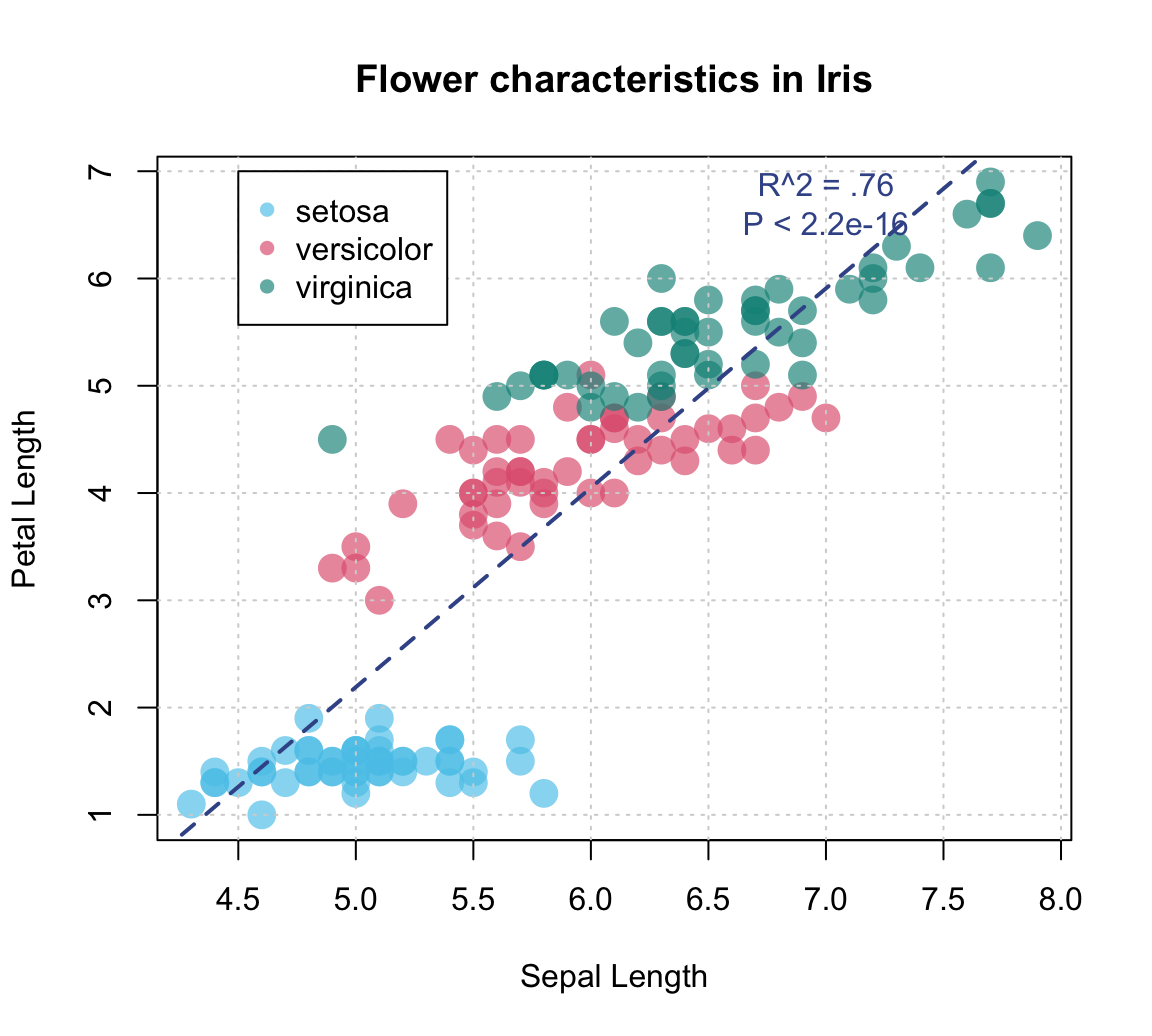An alternative (older) example: # Demo: Basic scatterplot (based on some data) with a regression line: # Create data: year <- ds4psy::what_year(ds4psy::sample_date(from = "1990-01-01", to = "2002-12-31", size = 100), as_integer = TRUE) value <- 2 * (year - 1990) + runif(length(year), 0, 100) df <- data.frame(year, value) # Scatterplot: plot(df$year, df$value, type = "p", col = "skyblue", lwd = 2, # aesthetic parameters xlab = "X label", ylab = "Y label", # axis labels main = "Line plot with regression line") # plot title # Regression: fit <- lm(value ~ year, data = df) # carry out linear regression fit ## ## Call: ## lm(formula = value ~ year, data = df) ## ## Coefficients: ## (Intercept) year ## -3867.288 1.971 abline(fit, lty = "dashed", col = "blue", lwd = 2) # add regression line # Adding text annotation: summary(fit) ## ## Call: ## lm(formula = value ~ year, data = df) ## ## Residuals: ## Min 1Q Median 3Q Max ## -53.255 -24.572 6.811 21.979 45.540 ## ## Coefficients: ## Estimate Std. Error t value Pr(>|t|) ## (Intercept) -3867.2876 1556.7758 -2.484 0.0147 * ## year 1.9707 0.7797 2.527 0.0131 * ## --- ## Signif. codes: 0 '***' 0.001 '**' 0.01 '*' 0.05 '.' 0.1 ' ' 1 ## ## Residual standard error: 28.8 on 98 degrees of freedom ## Multiple R-squared: 0.0612, Adjusted R-squared: 0.05162 ## F-statistic: 6.388 on 1 and 98 DF, p-value: 0.01309 text(x = 2000, y = 20, labels = "R^2 = 0.123\nP = 1.2e-12") # add a label to the plot at (x,y)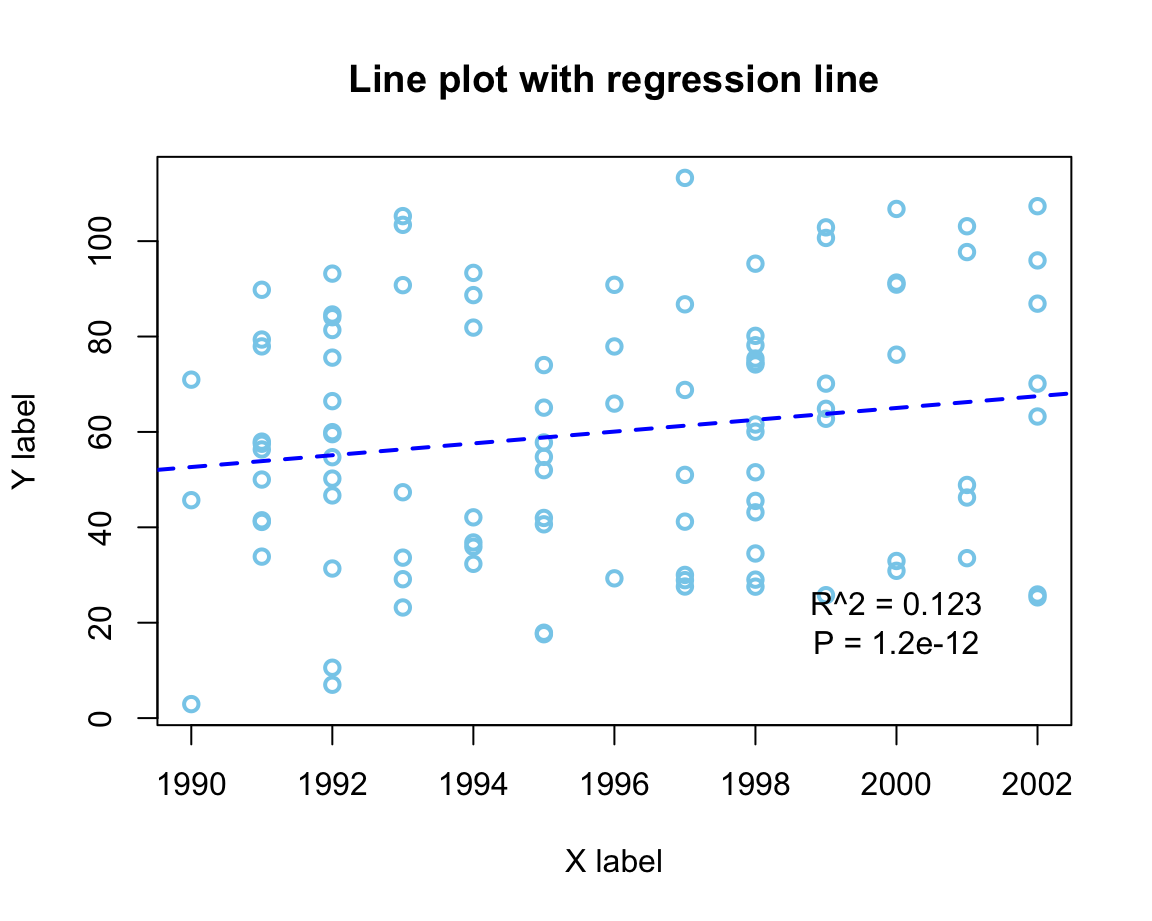Example 2: Plotting grouped raw data as a box plot: A scatterplot makes sense when both the x- and the y-dimension can be mapped to continuous variables. If one variable is continuous, but another one is categorical, the plot() function automatically chooses a box plot as a better alternative (see Figure ??). For instance, when using the mpg data to plot the values of the continuous variable cty as a function of the categorical variable class, we get: plot(cty ~ class, data = mpg, pch = 20, cex = 2, col = usecol(Seegruen, alpha = 1/4), main = "Fuel consumption in city by class of car")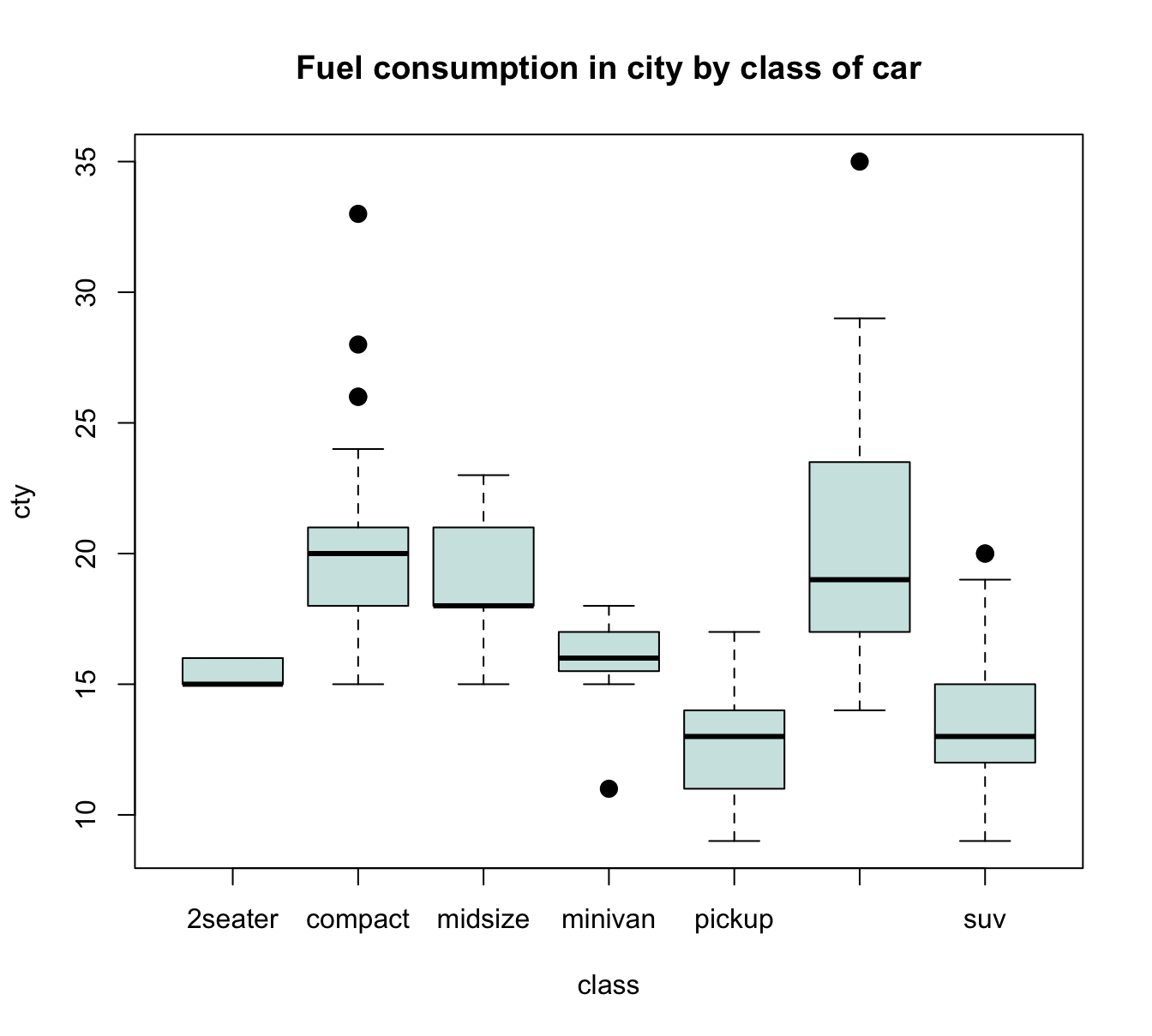Figure 4.5: A boxplot showing a continuous y-variable as a function of a categorical x-variable. Alternatively, it often is desirable to also see the individual data points. A means of doing so is provided by the jitter(x, factor) function, that accepts a numeric vector x and a factor or amount by which the values of the numeric vector are to be jittered (i.e., randomly increased and decreased). The idea behind jittering is that adding random noise can sometimes help to distinguish between elements (e.g., when plotting them). Jittering works best in combination with transparent colors, as this enables us to see the density of overlapping elements. In our present context, we primarily would need to add noise to our x-variable class, so that its values become distinguishable. Using jitter(as.numeric(class), 3/4) as our x-variable automatically transforms the boxplot into a scatterplot. However, we could also add some noise to our y-variable cty, which reduces the amount of overlap of points. Here are the results of both plots: plot(cty ~ jitter(as.numeric(class), 3/4), data = mpg, pch = 20, cex = 2, col = usecol(Bordeaux, alpha = 1/4), main = "A. cty by class (with horizontal jitter)") plot(jitter(cty, amount = 3/4) ~ jitter(as.numeric(class), 3/4), data = mpg, pch = 20, cex = 2, col = usecol(Bordeaux, alpha = 1/4), main = "B. cty by class (with horizontal and vertical jitter)")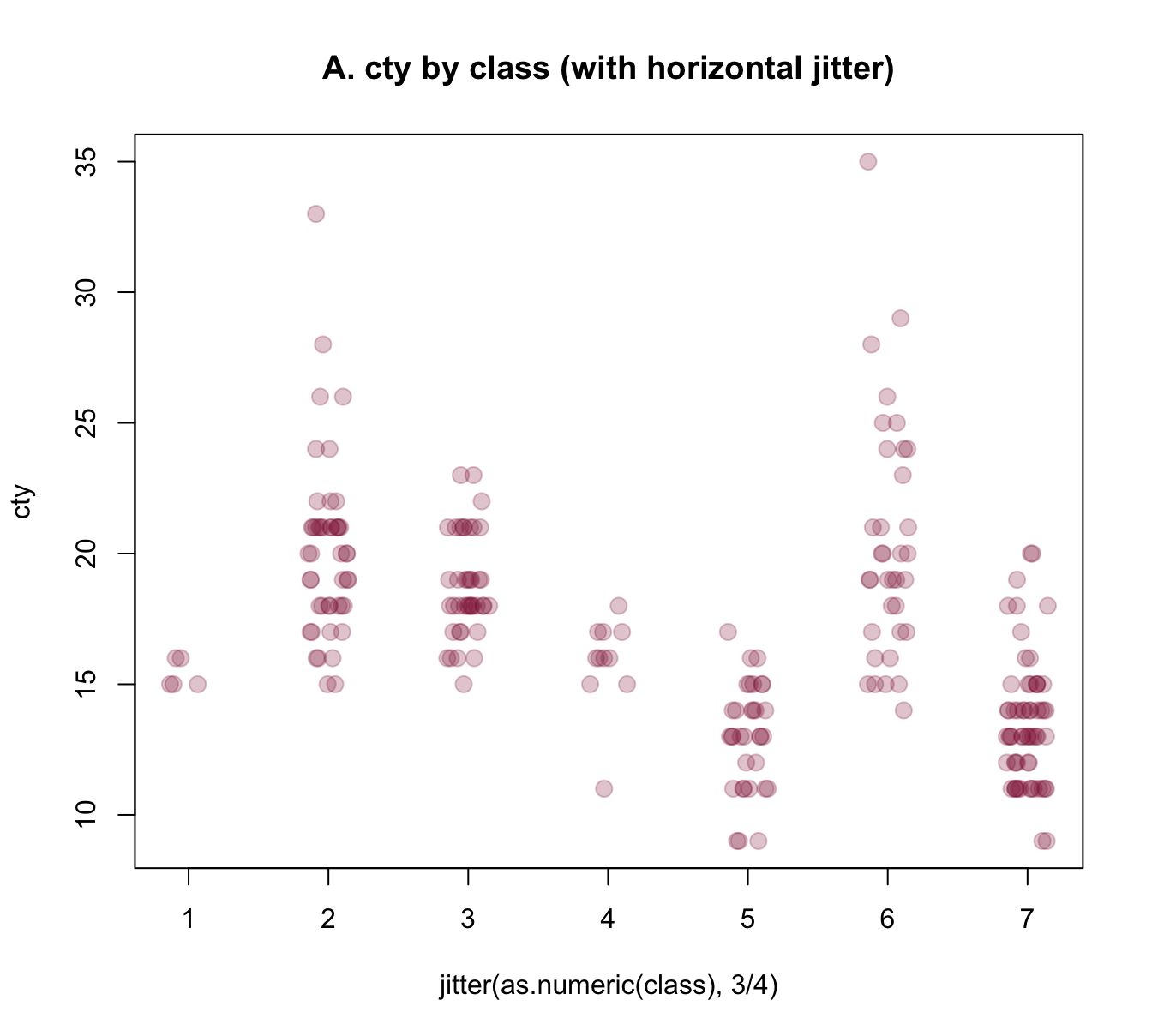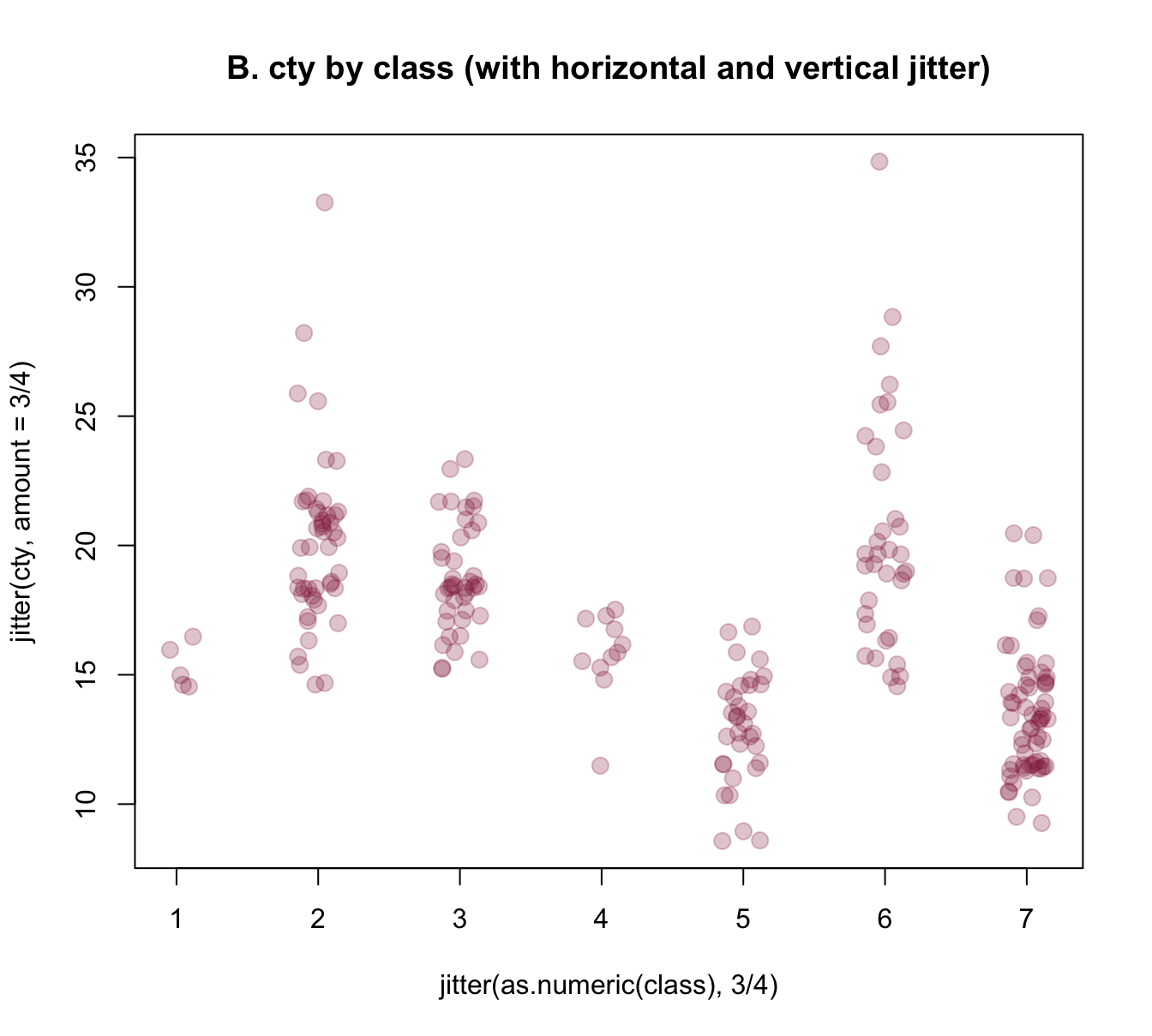Figure 4.6: Jittering categorical or/and continuous variables to show raw data points as scatterplots. The jittered raw data plots of Figure 4.6 provide some additional information over the boxplot of Figure 4.5 (e.g., they nicely illustrate the group sizes), but also have some deficiencies: 1. As we converted class into a numeric variable, their x-axis is numeric, rather than categorical. Can we use the previous class labels on our x-axis? 2. The raw data plots lack the explicit information about means and dispersion of groups that the boxplot provided. Can we combine both plots to provide a more complete picture? The answer to both questions is yes, of course. R provides several approaches for tackling these challenges, but all involve creating a plot in several steps. To gain more control about the axes, we could use an initial plot() function to define the basic dimensions and labels of our plot, but not yet include any axes (by setting axes = FALSE). We then add two axis() commands (to explicitly define an x- and y-axis and specify its steps, labels, and the orientation of labels) and a grid() command (to show some orientation lines in the background). Finally, we can use the points() annotation function with the jitter() instructions from above to add our raw data points to the canvas. Overall, the resulting plot provides a better impression of the raw data points: plot(x = 0, type = "n", xlim = c(0.5, 7), ylim = c(0, 40), axes = FALSE, main = "Raw data plot (with random jitter)", xlab = "Class X", ylab = "Value of Y") axis(1, at = 1:7, labels = levels(mpg$class), las = 2) # x-axis
axis(2, at = seq(0, 40, by = 5), las = 1) # y-axis

# plot raw data (as points with jitter):
points(jitter(cty, 3/4) ~ jitter(as.numeric(class), 3/4), data = mpg,
pch = 20, cex = 2,
col = usecol(Bordeaux, alpha = 1/4))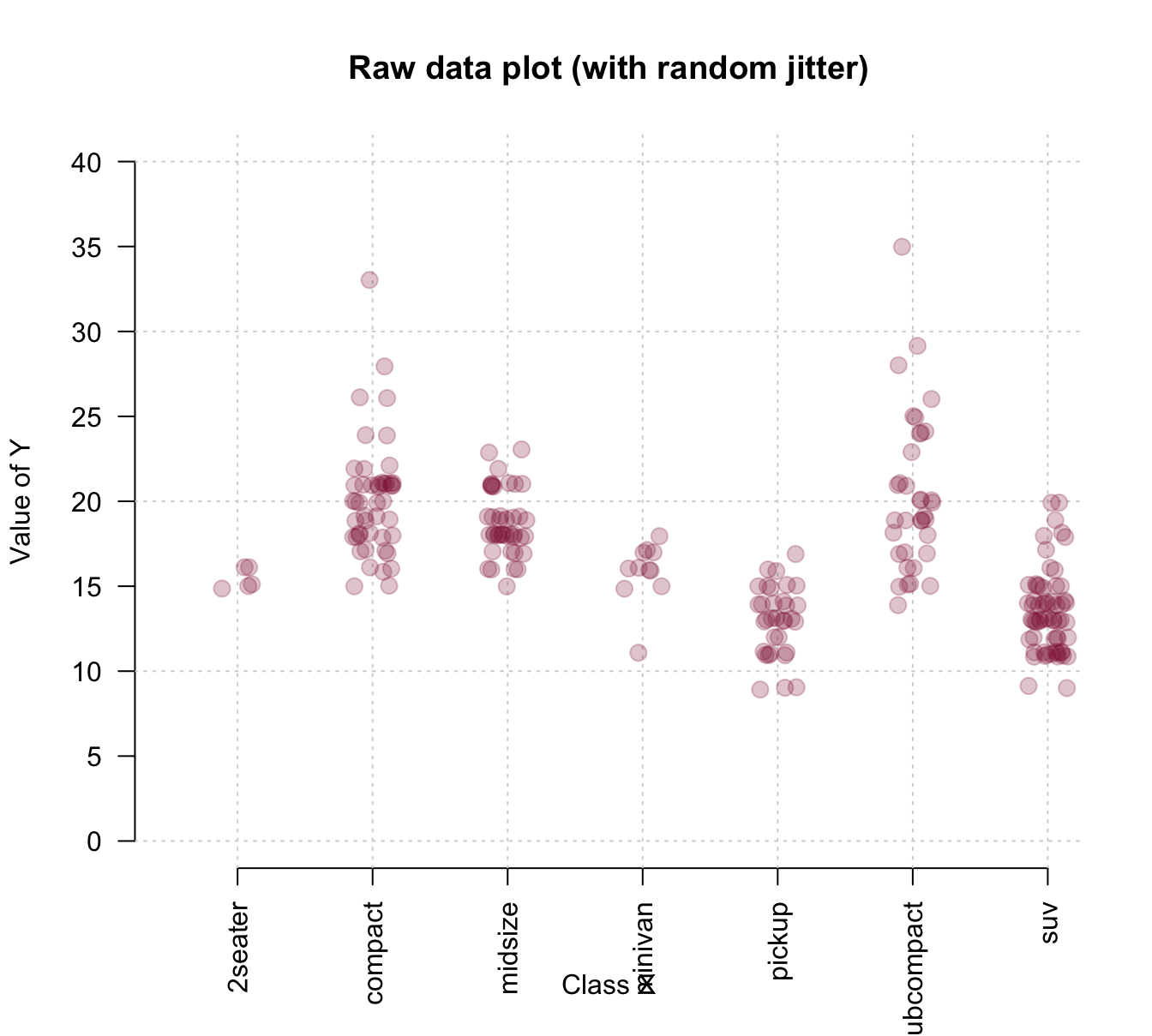To combine the benefits of the more abstract box plot with those of plotting the raw data, we could first draw a boxplot and later add the individual data points to it. Saving the boxplot to an R object also allows us to later use this object to add details to the plot. The following example inspects and uses the object bp to add text labels of group sizes and label two outliers:

# boxplot:
bp <- boxplot(cty ~ class, data = mpg,
col = usecol(Seegruen, alpha = 1/4),
las = 2, ylim = c(0, 40),
main = "Boxplot (with jittered raw data and some details)",
xlab = "Class of car", ylab = "MPG in city")

# plot raw data (as points with jitter):
points(jitter(cty, 3/4) ~ jitter(as.numeric(class), 3/4), data = mpg,
pch = 20, cex = 2,
col = usecol(Bordeaux, alpha = 1/4))

# Add group sizes (as text labels):
text(x = 1:7, y = 0, labels = paste0("[", bp$n, "]"), cex = .85) ## Check some outliers: # bp$out
# bp$group # mpg[mpg$cty>30, ]

text(x = 2, y = bp$out, labels = "VW New Beetle 1999", cex = .85, col = Bordeaux, pos = 3) text(x = 6, y = bp$out, labels = "VW Jetta 1999",
cex = .85, col = Bordeaux, pos = 3)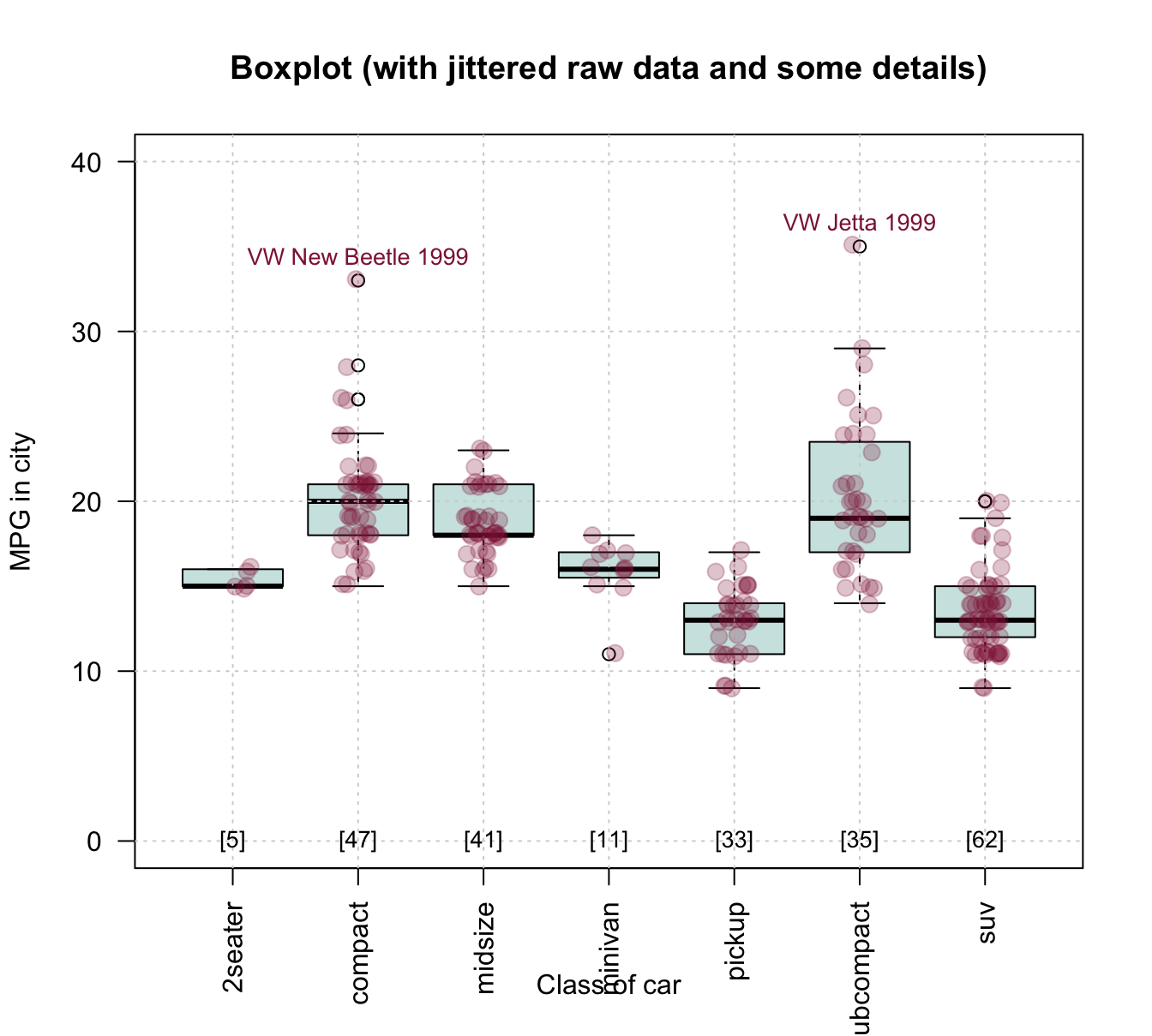Figure 4.7: Combining a box plot and a jittered raw data plot (plus some details).

Example 3: Plotting curves from functions:

Given some diagnostic test’s sens and spec values, what are the test’s positive and negative predictive values (PPV and NPV) as a function of prev?

Without understanding any details, we can use the functions provided by some package to compute the desired values. Here, we use the riskyr package (mentioned above, but not needed to create the visualizations of this section):

library(riskyr)

comp_PPV(prev = .05, sens = .99, spec = .95)
##  0.5103093
comp_NPV(prev = .05, sens = .99, spec = .95)
##  0.9994463

However, rather than just making predictions for individual prevalence values prev, we want a curve that shows PPV and NPV as a function of prev:

# Prepare canvas:
plot(x = 0, type = "n",
xlim = c(0, 1), ylim = c(0, 1),
main = "Predictive values as a function of prevalence",
xlab = "Prevalence", ylab = "Predictive values")

grid()

# Set parameters and compute some points:
prev <- .05
PPV  <- comp_PPV(prev = prev, sens = .99, spec = .95)
NPV  <- comp_NPV(prev = prev, sens = .99, spec = .95)

col_PPV <- "darkorange"
col_NPV <- "steelblue"

# Curves:
curve(expr = comp_PPV(prev = x, sens = .99, spec = .95),
from = 0, to = 1, col = col_PPV, lwd = 2, add = TRUE)

curve(expr = comp_NPV(prev = x, sens = .99, spec = .95),
from = 0, to = 1, col = col_NPV, lwd = 2, add = TRUE)

# Vertical line:
abline(v = prev, lty = 2, lwd = 1, col = "grey50")

# Points:
points(x = prev, y = PPV,
pch = 20, cex = 2, col = col_PPV)
points(x = prev, y = NPV,
pch = 20, cex = 2, col = col_NPV)

# Text labels:
text(x = prev, y = PPV, label = "PPV",
cex = 1, pos = 4, col = col_PPV)
text(x = prev, y = NPV, label = "NPV",
cex = 1, pos = 1, col = col_NPV)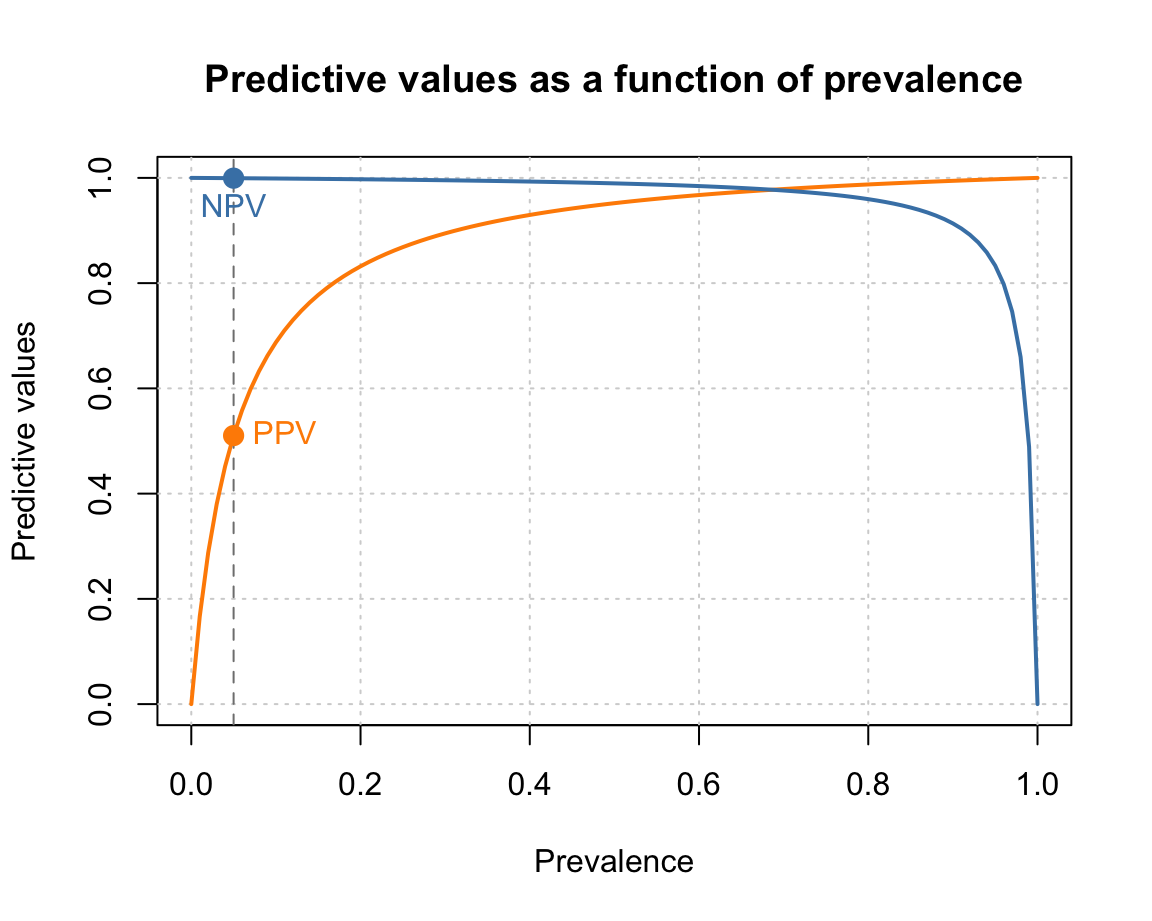Note that creating such plots implies many organizational and structural aspects. For instance, we first define some constants so that they can be used in various commands later. Similarly, the order of functions matters, as objects are plotted on top of earlier ones.

Overall, designing such plots implies writing a small script or computer program. Each step either defines some feature or computes an object by applying a function. When the steps are arranged and evaluated in the right order, they create a graphical representation.

+++ here now +++

#### Checking and setting graphical parameters (par())

The appearance of any graphical device in R is governed by many graphical parameters. The par() function allows checking and setting these parameters. It accepts a large number of parameters as tagged values (i.e., in a form tag = value, where tag is the name of a graphical parameter and value

• opar <- par() stores original (default) par settings (in an R object opar, short for “original par”)

Note: As some parameters can only be read, but not set, we typically exclude “read-only” parameters by storing opar <- par(no.readonly = TRUE).

• Setting par: par(mar = c(0, 0, 0, 0)) to remove margins

• par(opar) restores the original (default) par settings

See ?par for the list of parameters and options.

#### Arranging multiple plots

• Setting par(mfrow = c(1, 2))

#### Practice

1. Which graphical parameters can only be read, but not set?

Hint: Study the documentation of par(), or simply try storing and re-setting all_par <- par(); par(all_par).

### References

Neth, H., Gaisbauer, F., Gradwohl, N., & Gaissmaier, W. (2021). riskyr: Rendering risk literacy more transparent. https://riskyr.org/, https://CRAN.R-project.org/package=riskyr

1. However, remembering our notion of ecological rationality yields at least two other factors that matter for designing a good visualization: What is the message to be conveyed by the plot, and who will be viewing it?↩︎